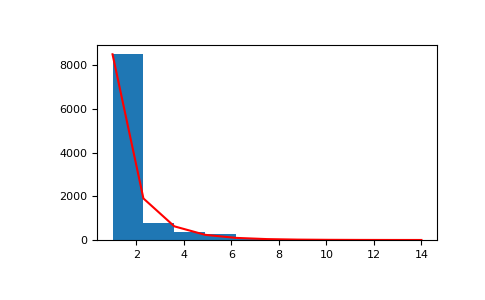# numpy.random.Generator.logseries¶

method

Generator.logseries(p, size=None)

Draw samples from a logarithmic series distribution.

Samples are drawn from a log series distribution with specified shape parameter, 0 < p < 1.

Parameters: p : float or array_like of floats Shape parameter for the distribution. Must be in the range (0, 1). size : int or tuple of ints, optional Output shape. If the given shape is, e.g., (m, n, k), then m * n * k samples are drawn. If size is None (default), a single value is returned if p is a scalar. Otherwise, np.array(p).size samples are drawn. out : ndarray or scalar Drawn samples from the parameterized logarithmic series distribution.

scipy.stats.logser
probability density function, distribution or cumulative density function, etc.

Notes

The probability mass function for the Log Series distribution iswhere p = probability.

The log series distribution is frequently used to represent species richness and occurrence, first proposed by Fisher, Corbet, and Williams in 1943 . It may also be used to model the numbers of occupants seen in cars .

References

  Buzas, Martin A.; Culver, Stephen J., Understanding regional species diversity through the log series distribution of occurrences: BIODIVERSITY RESEARCH Diversity & Distributions, Volume 5, Number 5, September 1999 , pp. 187-195(9).
  Fisher, R.A,, A.S. Corbet, and C.B. Williams. 1943. The relation between the number of species and the number of individuals in a random sample of an animal population. Journal of Animal Ecology, 12:42-58.
  D. J. Hand, F. Daly, D. Lunn, E. Ostrowski, A Handbook of Small Data Sets, CRC Press, 1994.
  Wikipedia, “Logarithmic distribution”, https://en.wikipedia.org/wiki/Logarithmic_distribution

Examples

Draw samples from the distribution:

>>> a = .6
>>> s = np.random.default_rng().logseries(a, 10000)
>>> import matplotlib.pyplot as plt
>>> count, bins, ignored = plt.hist(s)


# plot against distribution

>>> def logseries(k, p):
...     return -p**k/(k*np.log(1-p))
>>> plt.plot(bins, logseries(bins, a) * count.max()/
...          logseries(bins, a).max(), 'r')
>>> plt.show()#### Previous topic

numpy.random.Generator.lognormal

#### Next topic

numpy.random.Generator.multinomial